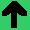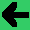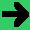# Lab 17: Utility

1. Write a program that calculates the utility of a bet that paid off 22% of the time 75Ł.
2. Now replace this with user input for one percentage and one utility value.
3. Now write a system that predicts the value of a 2-armed bandit problem.
4. Build the probabilities and values in. (Start with a constant value output. E.g 10% 50Ł vs. 90% 5Ł)
5. Run the system and keep track of the results.
6. Can your system pick the optimal move?
7. What happens if the values change?
8. If your system is trying to optimise expected value, does it ever pick a suboptimal outcome?